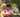### Missing smallest positive integer

January 23, 2020

Missing smallest positive integer

Write a function:
function solution(A);
that, given an array A of N integers, returns the smallest positive integer (greater than 0) that does not occur in A.

For example, given A = [1, 3, 6, 4, 1, 2], the function should return 5.
Given A = [1, 2, 3], the function should return 4.
Given A = [−1, −3], the function should return 1.

Write an efficient algorithm for the following assumptions:
• N is an integer within the range [1..100,000];
• each element of array A is an integer within the range [−1,000,000..1,000,000].

``````function solution(A) {
// write your code in JavaScript (Node.js 8.9.4)
let positive = []
let count = 0
for(let i=0; i<A.length; i++){
if(A[i]>0){
positive[ A[i] ] = true
count += 1
}
}
//console.log("count: ", count)
//console.log("positive.length: ", positive.length)
//non-assigned positive[] will be populated automatically
for(i=1; i<=positive.length; i++){ // = means that no missing in positive
if( ! positive[i] ) return i
}

return 1
}``````Posted by Tai Lu ( 呂台生 ) who's building useful things.You should follow him on Twitter